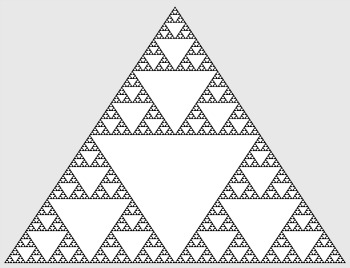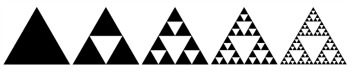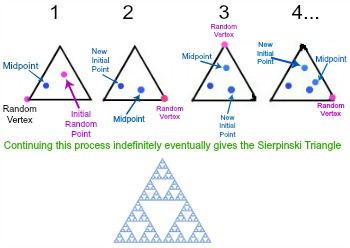# The Sierpinski Triangle & The Chaos Game

Instructor: Laura Pennington

Laura received her Master's degree in Pure Mathematics from Michigan State University, and her Bachelor's degree in Mathematics from Grand Valley State University. She has 20 years of experience teaching collegiate mathematics at various institutions.

This lesson will give an explanation of what the Sierpinski Triangle is and two different ways it can be constructed. We will also look at the connection between the chaos game and the construction of the Sierpinski Triangle.

## The Sierpinski Triangle

Suppose you're bored in class, and your eyes wander over to your neighbor's notes, and you see them doodling a really neat design as shown in the image.It just so happens that this result of boredom is actually a fascinating mathematical concept called the Sierpinski Triangle. The Sierpinski Triangle is a self-similar fractal…whoops, are you still with me? Let's first define a fractal and a self-similar shape before we get to the definition of the Sierpinski Triangle!

• Fractal - A fractal is a geometric construction that is created by repeatedly performing the same process or operation to a shape.
• Self-Similar Shapes - A self-similar shape is a shape that is similar to (or looks the same as) part of itself.

Ah, that's better! Okay, now let's try that again! The Sierpinski Triangle is a self-similar fractal that can be created quite simply using the following steps:

2. Find the midpoints of each of the sides of the triangle, and connect them using line segments. This will break the triangle up into four equal sized triangles. Remove the center triangle that you just created.
3. Do the same thing for the remaining three triangles within the original triangle.
4. Continue this process for each new triangle created indefinitely.That construction is pretty simple considering how detailed the end result looks! This actually isn't the only way to construct a Sierpinski Triangle. Another way involves the chaos game, and is a bit surprising. Let's explore!

## The Chaos Game and the Sierpinski Triangle

In mathematics, the chaos game is a manipulation of a polygon in such a way that a fractal is often, but not always, created. It involves starting with an initial random point within the polygon, and plotting points within the polygon that are a given fraction between vertices of the polygon and those points.

Using an equilateral triangle as the polygon, and a fraction of 1/2 will result in the Sierpinski Triangle. Huh, no wonder it's called the chaos game! That sounds a bit confusing! Let's step it out using an equilateral triangle and 1/2 as our fraction to better our understanding of the construction process and the chaos game itself. The steps we use in the chaos game to create a Sierpinski Triangle are as follows:

2. Choose one of the triangles vertices and a random point inside the triangle.
3. Plot the point that is midway between the vertex and random point.
4. Use the point you just plotted and one of the triangle's vertices and start again at step three.
5. Continue this process indefinitely.

This process must be done for quite a while, but believe it or not, after a long while of doing this, the triangle will look more and more like the Sierpinski Triangle.

## Example

Because of the fact that using the chaos game to construct the Sierpinski Triangle involves repeating the procedure an extremely large number of times for the triangle to really resemble the Sierpinski Triangle, computers are normally used for this type of construction. However, let's take a look at doing this for a few steps, so we can get a look at the chaos game and understand how the steps are performed better.To unlock this lesson you must be a Study.com Member.

### Register to view this lesson

Are you a student or a teacher?

Back

### Resources created by teachers for teachers

Over 30,000 video lessons & teaching resources‐all in one place.Video lessonsQuizzes & WorksheetsClassroom IntegrationLesson Plans

I would definitely recommend Study.com to my colleagues. It’s like a teacher waved a magic wand and did the work for me. I feel like it’s a lifeline.

Jennifer B.
TeacherCreate an account to start this course today
Used by over 30 million students worldwide